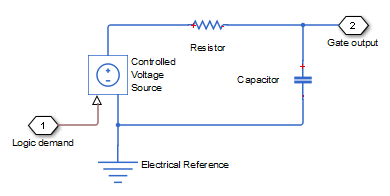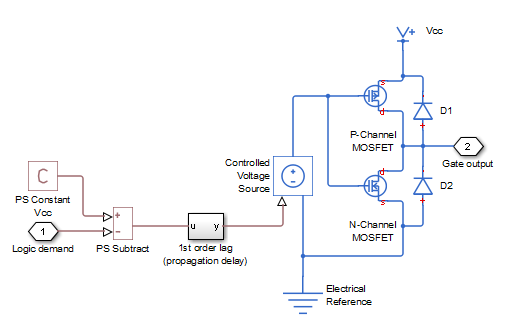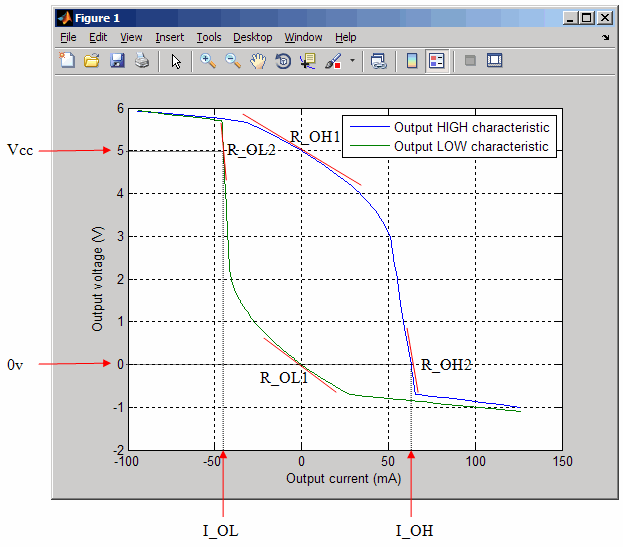## Selecting the Output Model for Logic Blocks

### Available Output Models

The blocks in the Logic sublibrary of the Integrated Circuits library provide a choice of two output models:

• `Linear` — Models the gate output as a voltage source driving a series resistor and capacitor connected to ground. This is suitable for logic circuit operation under normal conditions and when the logic gate drives other high-impedance CMOS gates. The block sets the value of the gate output capacitor such that the resistor-capacitor time constant equals the Propagation delay parameter value. The linear output model is shown in the following illustration.• `Quadratic` — Models the gate output in terms of a complementary N-channel and P-channel MOSFET pair. This adds more fidelity, which becomes relevant if drawing higher currents from the gate output, or if exercising the gate under fault conditions. In addition, the gate input demand is lagged to approximate the Propagation delay parameter value. Default parameters are representative of the 74HC logic gate family. The quadratic output model is shown in the next illustration.Use the Output current-voltage relationship parameter on the Outputs tab of the block dialog box to specify the output model.

For most system models, MathWorks recommends selecting the linear option because it supports faster simulation. If necessary, you can use the more detailed output model to validate simulation results obtained from the simpler model.

### Quadratic Model Output and Parameters

If you select the quadratic model, use the following parameters to control the block output:

• Supply voltage — Supply voltage value (Vcc) applied to the gate in your circuit. The default value is `5` V.

• Measurement voltage — The gate supply voltage for which mask data output resistances and currents are defined. The default value is `5` V.

• Logic HIGH output resistance at zero current and at I_OH — A row vector [ R_OH1 R_OH2 ] of two resistance values. The first value R_OH1 is the gradient of the output voltage-current relationship when the gate is logic HIGH and there is no output current. The second value R_OH2 is the gradient of the output voltage-current relationship when the gate is logic HIGH and the output current is I_OH. The default value is `[ 25 250 ]` Ω.

• Logic HIGH output current I_OH when shorted to ground — The resulting current when the gate is in the logic HIGH state, but the load forces the output voltage to zero. The default value is `63` mA.

• Logic LOW output resistance at zero current and at I_OL — A row vector [ R_OL1 R_OL2 ] of two resistance values. The first value R_OL1 is the gradient of the output voltage-current relationship when the gate is logic LOW and there is no output current. The second value R_OL2 is the gradient of the output voltage-current relationship when the gate is logic LOW and the output current is I_OL. The default value is `[ 30 800 ]` Ω.

• Logic LOW output current I_OL when shorted to Vcc — The resulting current when the gate is in the logic LOW state, but the load forces the output voltage to the supply voltage Vcc. The default value is `-45` mA.

• Propagation delay — Time it takes for the output to swing from `LOW` to `HIGH` or `HIGH` to `LOW` after the input logic levels change. For quadratic output, it is implemented by the lagged gate input demand. The default value is `25` ns.

• Protection diode on resistance — The gradient of the voltage-current relationship for the protection diodes when forward biased. The default value is `5` Ω.

• Protection diode forward voltage — The voltage above which the protection diode is turned on. The default value is `0.6` V.

The following graphic illustrates the quadratic output model parameterization, using the default parameter output characteristics for a +5V supply.